# Equations and Inequalities College Algebra Radical Equations Extraneous

• Slides: 22Equations and Inequalities College AlgebraExtraneous Solutions to Radical Equations •Rational Exponents •Polynomial Equations •Absolute Value Equations •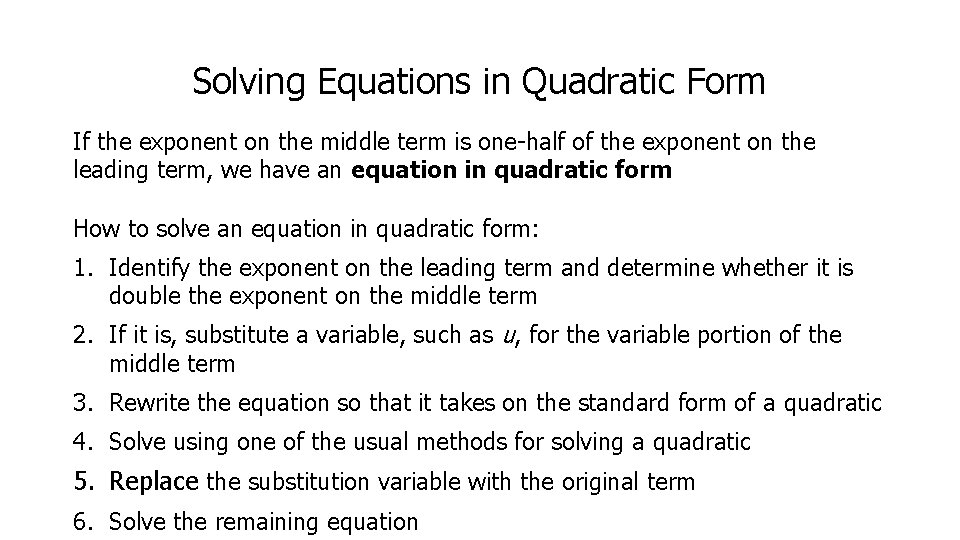Solving Equations in Quadratic Form If the exponent on the middle term is one-half of the exponent on the leading term, we have an equation in quadratic form How to solve an equation in quadratic form: 1. Identify the exponent on the leading term and determine whether it is double the exponent on the middle term 2. If it is, substitute a variable, such as u, for the variable portion of the middle term 3. Rewrite the equation so that it takes on the standard form of a quadratic 4. Solve using one of the usual methods for solving a quadratic 5. Replace the substitution variable with the original term 6. Solve the remaining equation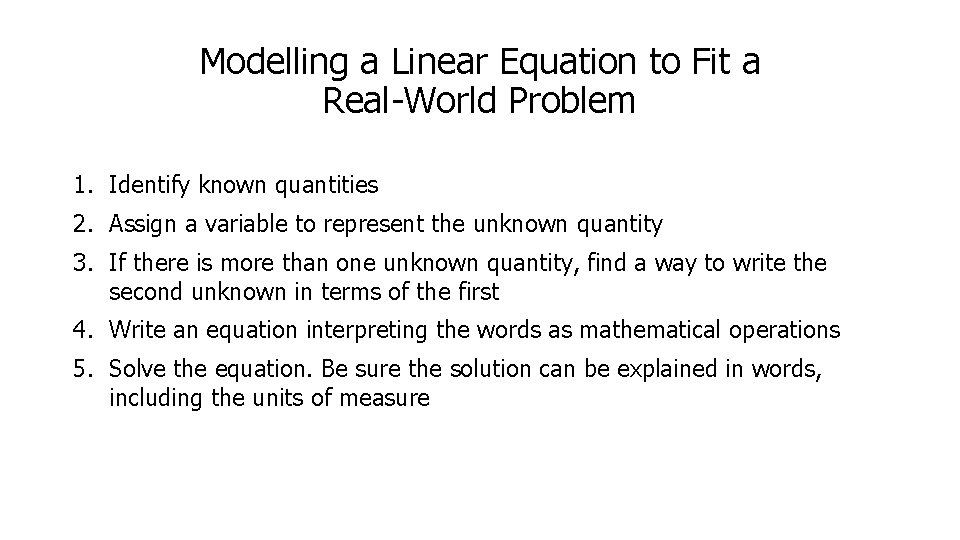Modelling a Linear Equation to Fit a Real-World Problem 1. Identify known quantities 2. Assign a variable to represent the unknown quantity 3. If there is more than one unknown quantity, find a way to write the second unknown in terms of the first 4. Write an equation interpreting the words as mathematical operations 5. Solve the equation. Be sure the solution can be explained in words, including the units of measure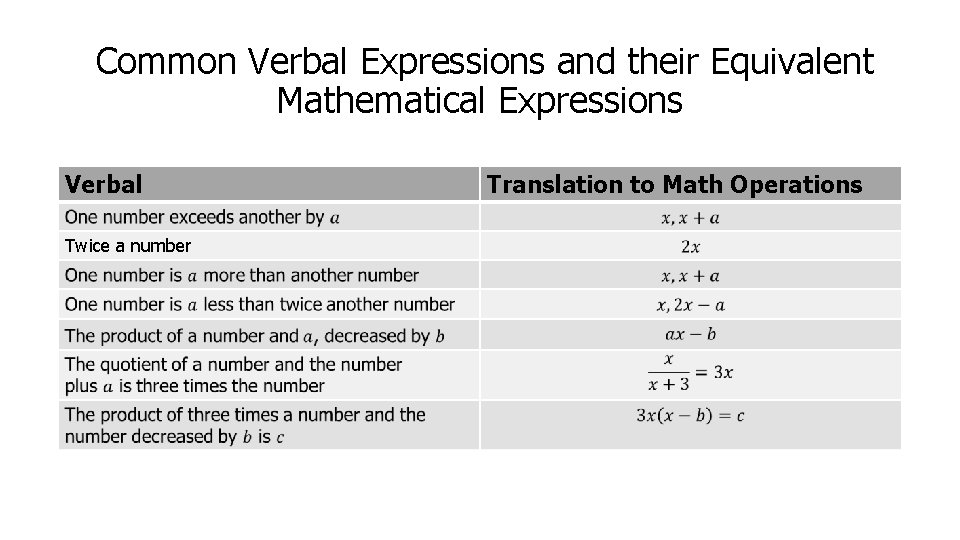Common Verbal Expressions and their Equivalent Mathematical Expressions Verbal Twice a number Translation to Math Operations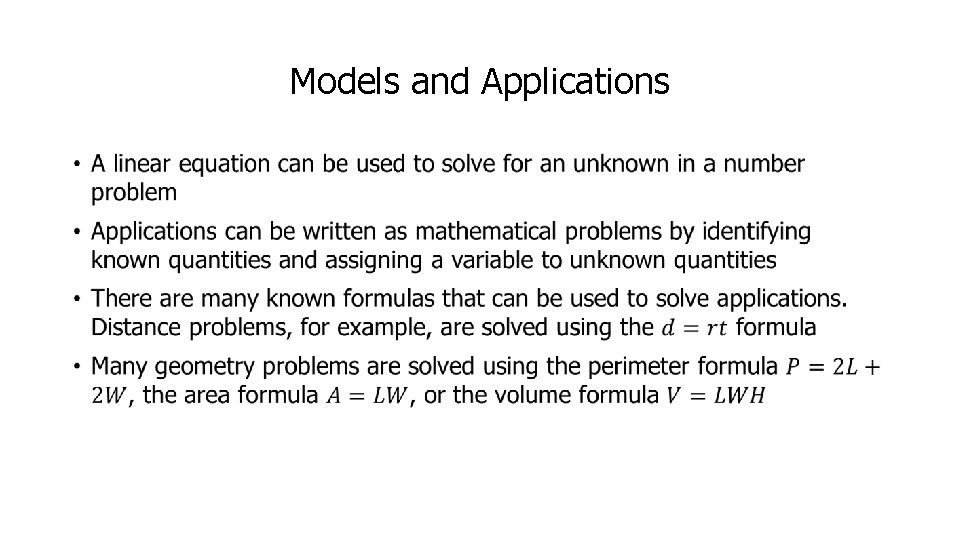Models and Applications •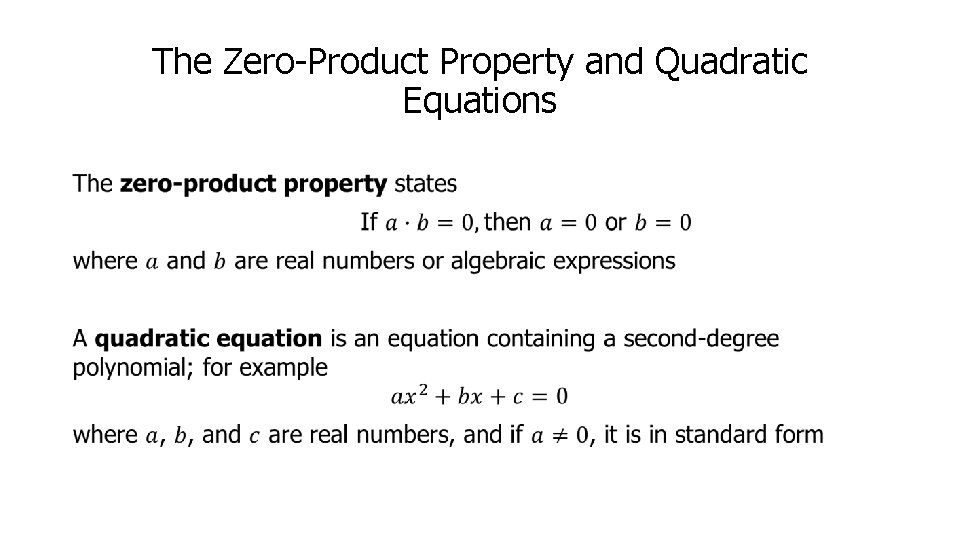The Zero-Product Property and Quadratic Equations •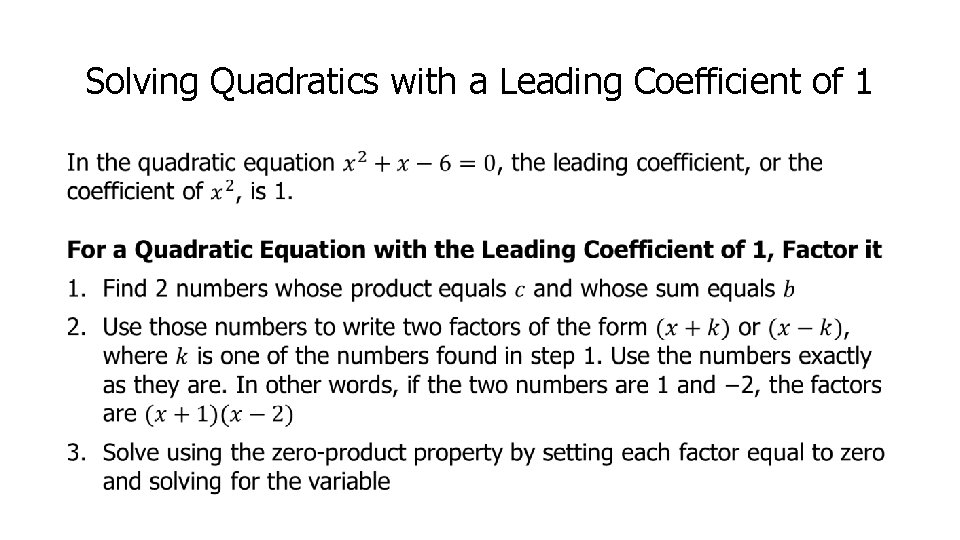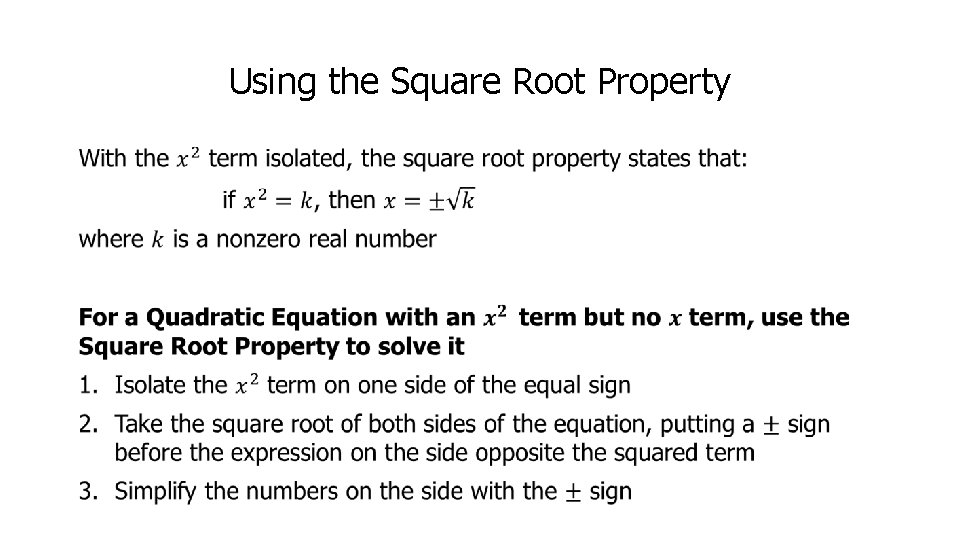Using the Square Root Property •Completing the Square •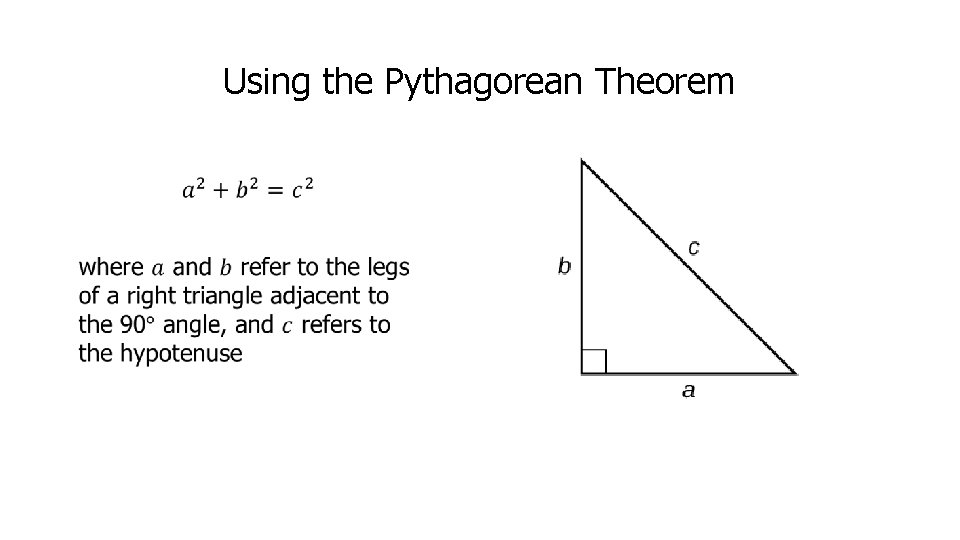Using the Pythagorean Theorem •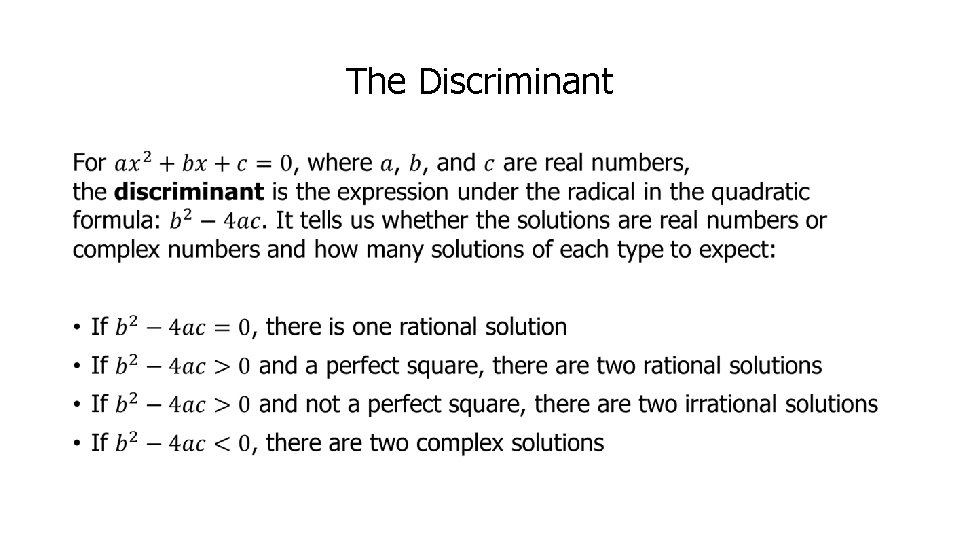The Discriminant •Set-Builder Notation and Interval Notation •The Properties of Inequalities •Desmos Interactive Topic: writing and graphing inequalities https: //www. desmos. com/calculator/4529 rytfefAbsolute Value of Inequalities •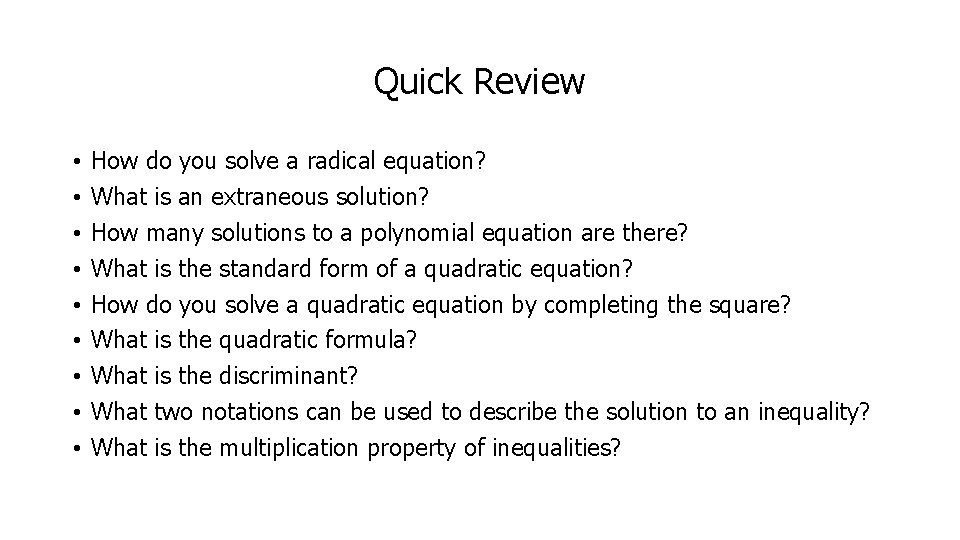Quick Review • • • How do you solve a radical equation? What is an extraneous solution? How many solutions to a polynomial equation are there? What is the standard form of a quadratic equation? How do you solve a quadratic equation by completing the square? What is the quadratic formula? What is the discriminant? What two notations can be used to describe the solution to an inequality? What is the multiplication property of inequalities?